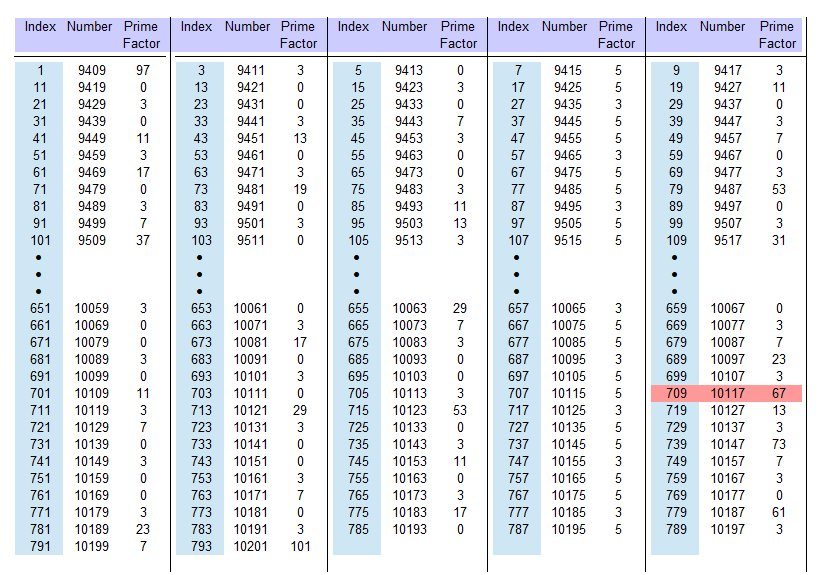# Primes and Prime Factors

## The Computation of a Prime Factor of 10117

In the following example, a prime divisor of the number 10117 is to be calculated. Let only the prime numbers < 49 = 72 be known. These prime numbers are stored in a file "FileP1.txt". The remainders or complements of the primes 2, 3, 5 and 7 at 49 are calculated. I have defined the complement as compi = pi − remainderi, i.e. the number 49 for example and pi = 5 then is 49 mod 5 = 4 and the next multiple of 5 is 50. Therefore here the complement is 1. The complements at 49 for the primes 2, 3, 5 and 7 are 1, 2, 1 and 7. The next prime is 11 and its square is 121. The difference 121 - 49 = 72 and therefore a fill area created for these elements has 73 elements. The even numbers are ignored. Therefore only the 1., 3., 5.,  . . . of the filling area calculated. This fill area is first emptied, i.e. all these elements are set to zero. Then the filling of the filling area beginsThe 3 has the complement 2 and fills the fields 3, 6, 9, . . . , the 5 has the complement 1 and fills the fields 2, 7, 12, . ..  . Accordingly the 7 fills the fields 8, 15, 22, . . . . After all fields are occupied, for the sake of completeness the first element with 7 and the last element with 11.

Now the evaluation of the filling area begins. All fields that have not been occupied correspond to the prime numbers in the range 49 to 121. They are stored in the file "FileP2.txt". The complements at 73 (in the filling range) or 121 are calculated. It can be obtained as follows: If the upper limit of 73 is exceeded by a multiple of a prime number during filling, then the complement results from the difference of this number - 73. With these complements start in the following fill aerea. To do this, the next prime number is read from "FileP1.txt". It is the 13. This filling range should accommodate the elements from 121 to 169 = 132. It has 49 elements. The elements of the fill area are set to zero and then with the help of the complements calculated at 121 the new fill area is filled. In addition to the complements of the numbers 3, 5, 7, the 11 is now added. It has the complement 11 (or 0) at 121. Again, the prime numbers result from the elements that have not been occupied. In the file "FileP2.txt" these prime numbers are added.

This procedure continues - initially until all prime numbers from "FileP1.txt" are used up. Figure 1 shows the squares of the prime numbers and the associated complements up to 472, i.e. up to 2209. Per line, the squares of the prime numbers and the complements of the prime numbers at these squares are listed. Now "FileP1.txt" is supplemented by the values from "FileP2.txt". After that, the file "FileP2.txt" is deleted and filled with the new prime numbers that are determined with the continuation of the procedure.

Table 1 lists the complements of the prime numbers in the squares of the prime numbers. The square 169 is smaller than the searched number 10177, which is to be broken down into prime dividers. Therefore, the next filling range is calculated. It is first emptied and filled with the prime numbers with the help of the complements. The resulting prime numbers are added to the prime numbers present in the "FileP2.txt" file. Continue with this method until all prime numbers from "FileP1.txt" are processed. This is the case with the prime number 47. Thus, the prime numbers from 53 to 2207, the largest prime number less than 472, are stored in file "FileP2.txt".Table 1; The squares of the prime numbers until 2209 and the complements of the prime numbers

If you continue in this way, you finally reach the number range in which pi2 ≤ 10117 ≤ pi+12. This is the range between 972 and 1012. Here, the filling area is evaluated more thoroughly. The result is shown in Table 2. Each element from the fill area corresponds to a number between pi2 and number range in which pi2 ≤ 10117 ≤ pi+12. This is the range between 972 and 1012. Here, the filling area is evaluated more thoroughly. The result is shown in Table 2. Each element from the fill area corresponds to a number between pi2 and pi+12.Table 2: The odd numbers from 9409 until 10201 and its lowest prime divisors

Only the ungraded numbers are listed. Under "Index" the index from the fill area is displayed. Under "Number" the respective number between the prime number squares is listed and with "Prime factor" a prime factor is displayed. It is the smallest prime factor of the corresponding number. As you can see, 10117 has a prime factor of 67. With a small change in the algorithm, the largest prime factor < pi+1 (here < 101) or all prime factors < 101 can also be displayed. Each factor is then listed only once. This algorithm has also been used to find the prime numbers up to 10201. The prime square and complements of the prime square 10201 are stored in a file. This has the advantage that you can use it when searching for a prime divider of a larger number can fall back on these values and does not have to start again at 49. Of course, you can save it anywhere (preferably with a square of a prime number).